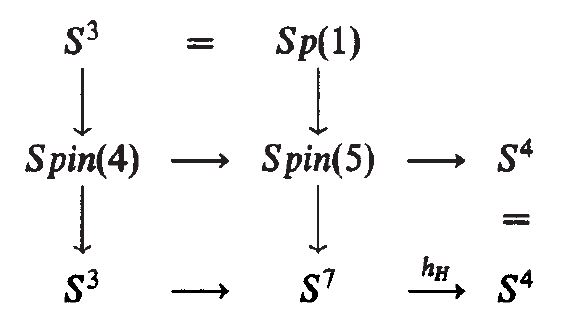# nLab Spin(5)

Contents

group theory

## Spin geometry

spin geometry

Dynkin labelsp. orth. groupspin grouppin groupsemi-spin group
SO(2)Spin(2)Pin(2)
B1SO(3)Spin(3)Pin(3)
D2SO(4)Spin(4)Pin(4)
B2SO(5)Spin(5)Pin(5)
D3SO(6)Spin(6)
B3SO(7)Spin(7)
D4SO(8)Spin(8)SO(8)
B4SO(9)Spin(9)
D5SO(10)Spin(10)
B5SO(11)Spin(11)
D6SO(12)Spin(12)
$\vdots$$\vdots$
D8SO(16)Spin(16)SemiSpin(16)
$\vdots$$\vdots$
D16SO(32)Spin(32)SemiSpin(32)

string geometry

# Contents

## Idea

The spin group in dimension 5.

## Properties

### Exceptional isomorphism

###### Proposition

There is an exceptional isomorphism

$Spin(5) \;\simeq\; Sp(2)$

between Spin(5) and the quaternionic unitary group $Sp(2) = U(2,\mathbb{H})$.

This is an indirect consequence of triality, see e.g. Čadek-Vanžura 97. Alternatively, it can be shown as follows.

Let $V$ be a 4-dimensional complex vector space with an inner product and a compatible complex volume form. As explained here, this structure can be used to define a conjugate-linear Hodge star operator on $\Lambda^2 V$ whose $+1$ and $-1$ eigenspaces, say $\Lambda_{\pm}^2 V$, are each 6-dimensional real inner product spaces. Thus, the group $\mathrm{SU}(V)$ acts as linear transformations of $\Lambda_{\pm}^2 V$ that preserve the inner product, giving a homomorphism $\rho: \mathrm{SU}(V) \to \mathrm{O}(\Lambda_{\pm}^2 V)$. In fact $\rho$ maps $\mathrm{SU}(V)$ in a 2-1 and onto way to $\mathrm{SO}(\Lambda_{\pm}^2 V)$. Taking $V = \mathbb{C}^4$ this shows $\mathrm{SU}(4) \cong \mathrm{Spin}(6)$.

Now suppose $V$ is additionally equipped with an complex symplectic structure, i.e. a nondegenerate skew-symmetric complex-bilinear form $J \in \Lambda^2 V$. The subgroup $\mathrm{Sp}(V)$ of $\mathrm{SU}(V)$ preserving this extra structure is isomorphic to the compact symplectic group $\mathrm{Sp}(2)$, which is also the quaternionic unitary group. This subgroup $\mathrm{Sp}(V)$ acts on $\Lambda_+^2 V$ and $\Lambda_-^2 V$ preserving $J \in \Lambda^2 V = \Lambda_+^2 V \oplus \Lambda_-^2 V$. Since $\mathrm{Sp}(V)$ is compact, every invariant subspace has an invariant complement, so one or both of the 6-dimensional subspaces $\Lambda_+^2 V$ and $\Lambda_-^2 V$ must have a 5-dimensional subspace invariant under the action of $\mathrm{Sp}(V)$. This shows that the double cover $\rho: \mathrm{SU}(4) \to \mathrm{SO}(6)$ restricts to a 2-1 homomorphism $\sigma : \mathrm{Sp}(2) \to \mathrm{SO}(5)$. Since

$\dim(\mathrm{Sp}(2)) = 10 = \dim(\mathrm{SO}(5))$

the differential $d\sigma$, being injective, must also be surjective. Thus $\sigma : \mathrm{Sp}(2) \to \mathrm{SO}(5)$ is actually a double cover. Since $\mathrm{Sp}(2)$ is connected this implies $\mathrm{Sp}(2) \cong \mathrm{Spin}(5)$.

### Action on quaternionic Hopf fibration

###### Proposition

(Spin(5)-equivariance of quaternionic Hopf fibration)

Consider

1. the Spin(5)-action on the 4-sphere $S^4$ which is induced by the defining action on $\mathbb{R}^5$ under the identification $S^4 \simeq S(\mathbb{R}^5)$;

2. the Spin(5)-action on the 7-sphere $S^7$ which is induced under the exceptional isomorphism $Spin(5) \simeq Sp(2) = U(2,\mathbb{H})$ (from Prop. ) by the canonical left action of $U(2,\mathbb{H})$ on $\mathbb{H}^2$ via $S^7 \simeq S(\mathbb{H}^2)$.

Then the quaternionic Hopf fibration $S^7 \overset{h_{\mathbb{H}}}{\longrightarrow} S^4$ is equivariant with respect to these actions.

This is almost explicit in Porteous 95, p. 263### Cohomology

###### Proposition

The integral cohomology ring of the classifying space $B Spin(5)$ is spanned by two generators

1. the first fractional Pontryagin class $\tfrac{1}{2}p_1$

2. the linear combination $\tfrac{1}{2}p_2 - \tfrac{1}{2}(p_1)^2$ of the half the second Pontryagin class with half the cup product-square of the first Pontryagin class:

$H^\bullet \big( B Spin(5), \mathbb{Z} \big) \;\simeq\; \mathbb{Z} \left[ \tfrac{1}{2}p_1, \; \tfrac{1}{2}p_2 - \tfrac{1}{2}(p_1)^2 \right]$

This is a special case of the general statement in Pittie 91, see e.g. Kalkkinen 06, Section 3).

###### Proposition

Let

$\array{ S^4 &\longrightarrow& B Spin(4) \\ && \big\downarrow^{\mathrlap{\pi}} \\ && B Spin(5) }$

be the spherical fibration of classifying spaces induced from the canonical inclusion of Spin(4) into Spin(5) and using that the 4-sphere is equivalently the coset space $S^4 \simeq Spin(5)/Spin(4)$ (this Prop.).

Then the fiber integration of the odd cup powers $\chi^{2k+1}$ of the Euler class $\chi \in H^4\big( B Spin(4), \mathbb{Z}\big)$ (see this Prop) are proportional to cup powers of the second Pontryagin class

$\pi_\ast \left( \chi^{2k+1} \right) \;=\; 2 \big( p_2 \big)^k \;\;\in\;\; H^4\big( B Spin(5), \mathbb{Z} \big) \,,$

for instance

\begin{aligned} \pi_\ast \big( \chi \big) & = 2 \\ \pi_\ast \left( \chi^3 \right) & = 2 p_2 \\ \pi_\ast \left( \chi^5 \right) & = 2 (p_2)^2 \end{aligned} \;\;\in\;\; H^4\big( B Spin(5), \mathbb{Z} \big) \,;

while the fiber integration of the even cup powers $\chi^{2k}$ vanishes

$\pi_\ast \left( \chi^{2k} \right) \;=\; 0 \;\;\in\;\; H^4\big( B Spin(5), \mathbb{Z} \big) \,.$

### Coset spaces

coset space-structures on n-spheres:

standard:
$S^{n-1} \simeq_{diff} SO(n)/SO(n-1)$this Prop.
$S^{2n-1} \simeq_{diff} SU(n)/SU(n-1)$this Prop.
$S^{4n-1} \simeq_{diff} Sp(n)/Sp(n-1)$this Prop.
exceptional:
$S^7 \simeq_{diff} Spin(7)/G_2$Spin(7)/G2 is the 7-sphere
$S^7 \simeq_{diff} Spin(6)/SU(3)$since Spin(6) $\simeq$ SU(4)
$S^7 \simeq_{diff} Spin(5)/SU(2)$since Sp(2) is Spin(5) and Sp(1) is SU(2), see Spin(5)/SU(2) is the 7-sphere
$S^6 \simeq_{diff} G_2/SU(3)$G2/SU(3) is the 6-sphere
$S^15 \simeq_{diff} Spin(9)/Spin(7)$Spin(9)/Spin(7) is the 15-sphere(from FSS 19, 3.4)

### $G$-Structure and exceptional geometry

Spin(8)-subgroups and reductions to exceptional geometry

reductionfrom spin groupto maximal subgroup
Spin(7)-structureSpin(8)Spin(7)
G2-structureSpin(7)G2
CY3-structureSpin(6)SU(3)
SU(2)-structureSpin(5)SU(2)
generalized reductionfrom Narain groupto direct product group
generalized Spin(7)-structure$Spin(8,8)$$Spin(7) \times Spin(7)$
generalized G2-structure$Spin(7,7)$$G_2 \times G_2$
generalized CY3$Spin(6,6)$$SU(3) \times SU(3)$

Dynkin labelsp. orth. groupspin grouppin groupsemi-spin group
SO(2)Spin(2)Pin(2)
B1SO(3)Spin(3)Pin(3)
D2SO(4)Spin(4)Pin(4)
B2SO(5)Spin(5)Pin(5)
D3SO(6)Spin(6)
B3SO(7)Spin(7)
D4SO(8)Spin(8)SO(8)
B4SO(9)Spin(9)
D5SO(10)Spin(10)
B5SO(11)Spin(11)
D6SO(12)Spin(12)
$\vdots$$\vdots$
D8SO(16)Spin(16)SemiSpin(16)
$\vdots$$\vdots$
D16SO(32)Spin(32)SemiSpin(32)